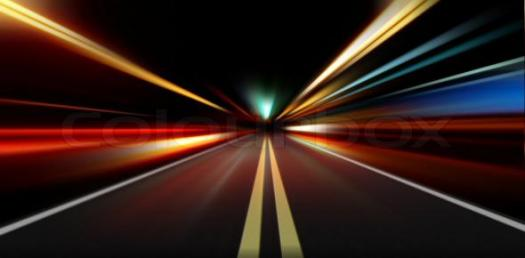# Speed And Acceleration Trivia Quiz

10 Questions | Attempts: 251SettingsSpeed is the rate at which a distance is covered per time. In other words, it is the rate at which an object includes distance. Acceleration defined as the rate of change of velocity of an object with respect to the time taken. An object's acceleration is known by the total result of all forces acting on the object, as described by Newton's Second Law. Are you familiar with these terms in Physics? Try this quiz out.Back to top